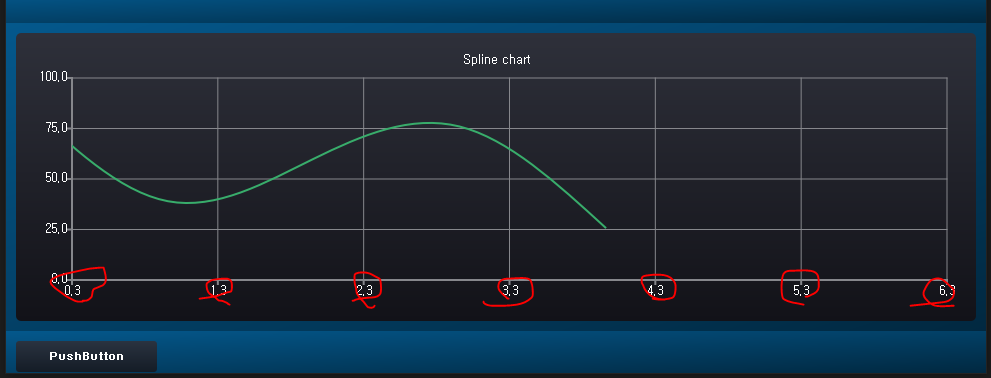# Spline Chart

•``````QChart* QTemperatureChart::createSplineChart()
{
m_pChart = new QChart();
m_pChart->setTitle("Spline chart");

// The lower series initialized to zero values
QString name("Series ");
int nameIndex = 0;

m_pSplineSeries = new QSplineSeries(m_pChart);

m_pSplineSeries->attachAxis(m_valueAxisX);
m_pSplineSeries->attachAxis(m_valueAxisY);

m_valueAxisX->setTickCount(7);
m_valueAxisX->setRange(0, 6);
m_valueAxisY->setRange(0, 100);

//m_pChart->createDefaultAxes();
//m_pChart->axes(Qt::Vertical).first()->setRange(0, 10);

return m_pChart;
}

void QTemperatureChart::UpdateChart(QPointF point)
{
int randomx = QRandomGenerator::global()->bounded(0, 100);
int randomy = QRandomGenerator::global()->bounded(0, 100);

m_pSplineSeries->append(m_pSplineSeries->count(), randomy);
m_pChart->scroll(10, 0);
}
``````I want to increase the x-axis by one on the chart, but it's increasing by 0.1.
And I want to fix the graph in the middle, so I think I can use Scroll.
But it doesn't work.